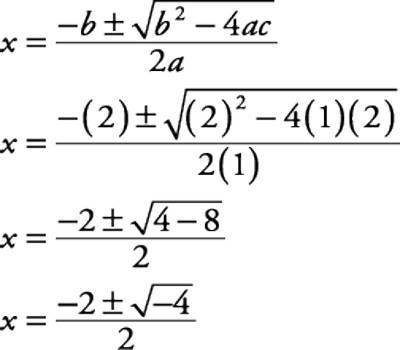A polynomial of the second degree is generally called a quadratic polynomial. In elementary algebra, the quadratic formula is the solution of the quadratic equation. There are other ways to solve the quadratic equation instead of using the quadratic formula, such as factoring, completing the square, or graphing. Using the quadratic formula is often the most convenient way.

If f(x) is a quadratic polynomial, then f(x) = 0 is called a quadratic equation.

An equation in one unknown quantity in the form ax2 + bx + c = 0 is called quadratic equation.

A quadratic equation is an equation of the second degree.

Nature of the Roots of a Quadratic Equation

We know that α and β are the roots of the general form of the quadratic equation ax2 + bx + c = 0 (a ≠ 0) ……………….. (i) then we get

α = [− b − √(b2 −4ac)] / 2a and β = [−b + √(b2 − 4ac)] / 2a

Here a, b and c are real and rational.

Then, the nature of the roots α and β of equation ax2 + bx + c = 0 depends on the quantity or expression i.e., (b2 – 4ac) under the square root sign.

Thus the expression (b2 – 4ac) is called the discriminant of the quadratic equation ax2 + bx + c = 0.

Generally, we denote discriminant of the quadratic equation by ‘∆ ‘ or ‘D’.

Therefore,

Discriminant ∆ = b2 – 4ac

Depending on the discriminant we shall discuss the following cases about the nature of roots α and β of the quadratic equation ax2 + bx + c = 0.

When a, b and c are real numbers, a ≠ 0Case I: b – 4ac > 0

When a, b and c are real numbers, a ≠ 0 and discriminant is positive (i.e., b2 – 4ac > 0), then the roots α and β of the quadratic equation ax2 + bx + c = 0 are real and unequal.

Case II: b2 – 4ac = 0

When a, b and c are real numbers, a ≠ 0 and discriminant is zero (i.e., b2 – 4ac = 0), then the roots α and β of the quadratic equation ax2 + bx + c = 0 are real and equal.

Case III: b2 – 4ac < 0

When a, b and c are real numbers, a ≠ 0 and discriminant is negative (i.e., b2 – 4ac < 0), then the roots α and β of the quadratic equation ax2 + bx + c = 0 are unequal and imaginary. Here the roots α and β are a pair of the complex conjugates.

Case IV: b2 – 4ac > 0 and perfect square

When a, b and c are real numbers, a ≠ 0 and discriminant is positive and perfect square, then the roots α and β of the quadratic equation ax2 + bx + c = 0 are real, rational unequal.

Case V: b2 – 4ac > 0 and not perfect square

When a, b and c are real numbers, a ≠ 0 and discriminant is positive but not a perfect square then the roots of the quadratic equation ax2 + bx + c = 0 are real, irrational and unequal.

Here the roots α and β form a pair of irrational conjugates.

Case VI: b2 – 4ac is perfect square and a or b is irrational

When a, b and c are real numbers, a ≠ 0 and the discriminant is a perfect square but any one of a or b is irrational than the roots of the quadratic equation ax2 + bx + c = 0 are irrational.

Notes:

(i) From Case I and Case II we conclude that the roots of the quadratic equation ax2 + bx + c = 0 are real when b2 – 4ac ≥ 0 or b2 – 4ac ≮ 0.

(ii) From Case I, Case IV and Case V we conclude that the quadratic equation with real coefficient cannot have one real and one imaginary root; either both the roots are real when b2 – 4ac > 0 or both the roots are imaginary when b2 – 4ac < 0.

(iii) From Case IV and Case V, we conclude that the quadratic equation with rational coefficient cannot have only one rational and only one irrational root; either both the roots are rational when b2 – 4ac is a perfect square or both the roots are irrational b2 – 4ac is not a perfect square.

Information Source: